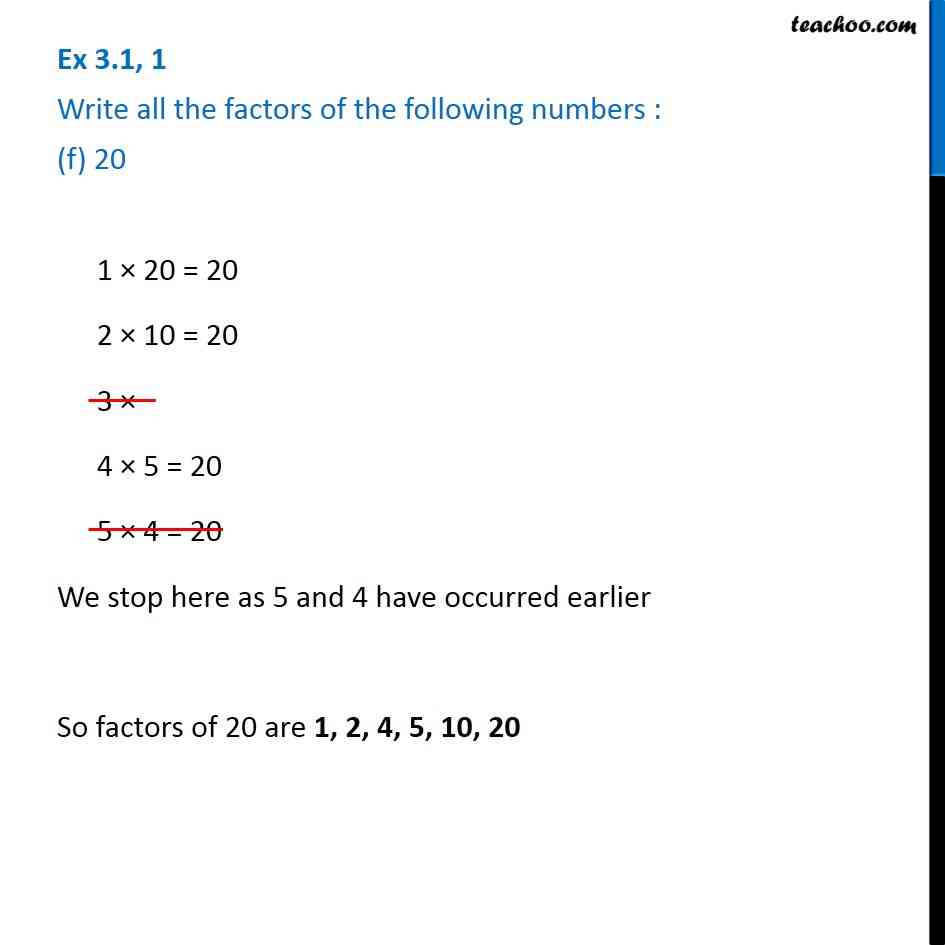1. Chapter 3 Class 6 Playing with Numbers
2. Serial order wise
3. Ex 3.1

Transcript

Ex 3.1, 1 Write all the factors of the following numbers : (f) 20 1 × 20 = 20 2 × 10 = 20 3 × 4 × 5 = 20 5 × 4 = 20 We stop here as 5 and 4 have occurred earlier So factors of 20 are 1, 2, 4, 5, 10, 20